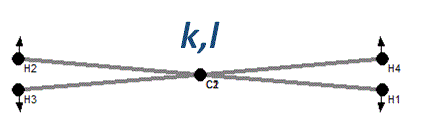Molecule Types Vibrational Structure Force Field Analysis

# Valence Coordinates

The valence coordinates are closely based on those described in L. Hedberg, I. M. Mills,  J. Molec. Spectrosc. 160, 117 (1993), and this should be referred to for precise definitions and units. Any differences are noted below.

The units of the coordinates are Å for the stretches and radians for the others (all some form of angle), so the stretching force constants have different units to the others.

### Stretch

Bond length between atom k and l: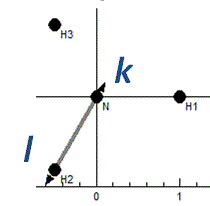### Bend

The angle klm: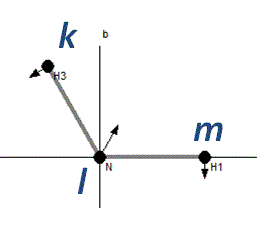### Linear Bend

For atoms in a line an additional constraint is required, and four atoms must be specified rather than three. (The name is still bend in the  internal coordinates object.) In contrast to the Hedberg and Mills paper the coordinate is taken to be the angle klm in plane of atom n, rather than specifying a separate vector. The extra atom, n, will typically be a dummy atom. (If the atoms are not in a line an additional atom can still be specified; formally the displacements are then parallel to the plane containing k l and n.)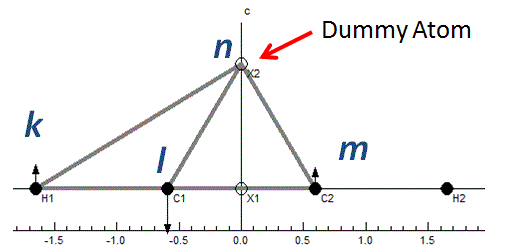### BondPlane

This is type 2 out of plane bending in the nomenclature of Hedberg and Mills. It is the angle between bond kl and plane mkn. The plane is indicated by a triangle in the plot: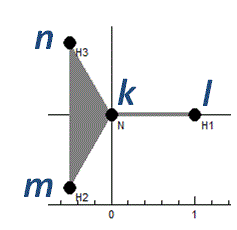and the side projection shows the motion: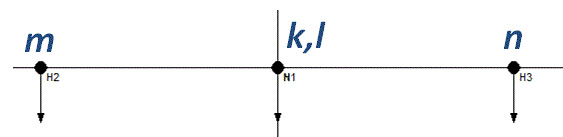### Plane

This is type 1 out of plane bending in the nomenclature of Hedberg and Mills. Given centre (or apex) atom k and three other atoms in the same plane (lmn) the coordinate is the scalar triple product of unit vectors along bonds:
eklekm × ekn.
All the atoms are shown joined to the central atom:and again a different projection is needed to show the motion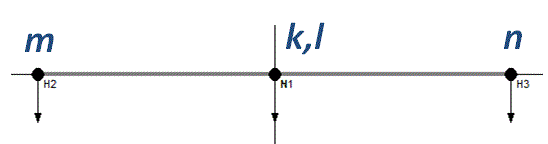### Torsion

Given atoms klmn in a chain, the coordinate is the angle between the planes klm and lmn. (The atoms klm and lmn must not be collinear.) The chain is shown in the plot window:and a different projection shows the displacements: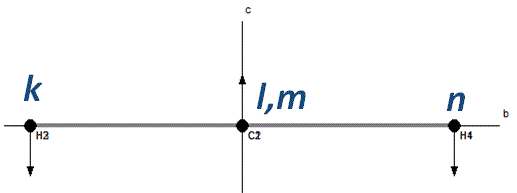### Torsion (Generalised)

In an extension to the torsion as defined by Hedberg and Mills above, a generalised torsion can be given by only specifying two (bonded) atoms, kl for a torsion. The valence coordinate is then an average of all possible 4 atom torsions defined as above, identifying the bonded atoms from all the other valence coordinates. (All the bonds indicated above are counted, apart from the 4th atom in the linear bend.) The plot window joins all the affected atoms:and again a different projection shows the displacements: